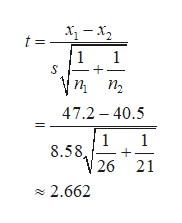# Use the given statistics to complete parts​ (a) and​ (b). Assume that the populations are normally distributed.​(a) Test whethermu 1μ1greater than>mu 2μ2at thealphaαequals=0.05level of significance for the given sample data.​(b) Construct a90​%confidence interval aboutmu 1μ1minus−mu 2μ2.  Population 1Population 2 n2621x overbarx47.240.5s4.611.8​(a) Identify the null and alternative hypotheses for this test.  A.Upper H 0H0​:mu 1μ1less than<mu 2μ2Upper H 1H1​:mu 1μ1equals=mu 2μ2 B.Upper H 0H0​:mu 1μ1equals=mu 2μ2Upper H 1H1​:mu 1μ1not equals≠mu 2μ2 C.Upper H 0H0​:mu 1μ1not equals≠mu 2μ2Upper H 1H1​:mu 1μ1equals=mu 2μ2 D.Upper H 0H0​:mu 1μ1greater than>mu 2μ2Upper H 1H1​:mu 1μ1equals=mu 2μ2 E.Upper H 0H0​:mu 1μ1equals=mu 2μ2Upper H 1H1​:mu 1μ1greater than>mu 2μ2Your answer is correct. F.Upper H 0H0​:mu 1μ1equals=mu 2μ2Upper H 1H1​:mu 1μ1less than<mu 2μ2 Find the test statistic for this hypothesis test. nothing​(Round to two decimal places as​ needed.)

Question
24 views

 Population 1 Population 2 Use the given statistics to complete parts​ (a) and​ (b). Assume that the populations are normally distributed. ​(a) Test whether mu 1μ1greater than>mu 2μ2 at the alphaαequals=0.05 level of significance for the given sample data. ​(b) Construct a 90​% confidence interval about mu 1μ1minus−mu 2μ2. 26 21 47.2 40.5 4.6 11.8
​(a) Identify the null and alternative hypotheses for this test.

A.
Upper H 0H0​:
mu 1μ1less than<mu 2μ2
Upper H 1H1​:
mu 1μ1equals=mu 2μ2

B.
Upper H 0H0​:
mu 1μ1equals=mu 2μ2
Upper H 1H1​:
mu 1μ1not equals≠mu 2μ2

C.
Upper H 0H0​:
mu 1μ1not equals≠mu 2μ2
Upper H 1H1​:
mu 1μ1equals=mu 2μ2

D.
Upper H 0H0​:
mu 1μ1greater than>mu 2μ2
Upper H 1H1​:
mu 1μ1equals=mu 2μ2

E.
Upper H 0H0​:
mu 1μ1equals=mu 2μ2
Upper H 1H1​:
mu 1μ1greater than>mu 2μ2

F.
Upper H 0H0​:
mu 1μ1equals=mu 2μ2
Upper H 1H1​:
mu 1μ1less than<mu 2μ2

Find the test statistic for this hypothesis test.

nothing
​(Round to two decimal places as​ needed.)
check_circle

Step 1

Hypotheses for the test is given below:

The correct answer is option E.

Step 2

This test is a right-tailed test.

Test statistic for t-test:

Since population standard deviations are unknown, the appropriate test is two sample t-test.

Here, Sample sizes of 1st and 2nd samples are 26 and 21 respectively.

The sample means of 1st and 2nd samples are 47.2 and 40.5 respectively.

Sample standard deviations of 1st and 2nd samples are 4.6 and 11.8 respectively.

The pooled standard deviation can be calculated as follows:

Step 3

The test statistic for t-test is cal...help_outlineImage Transcriptioncloset = 1 1 п п, 47.2 40.5 1 1 8.58 26 21 2.662 fullscreen

### Want to see the full answer?

See Solution

#### Want to see this answer and more?

Solutions are written by subject experts who are available 24/7. Questions are typically answered within 1 hour.*

See Solution
*Response times may vary by subject and question.
Tagged in

### Hypothesis Testing